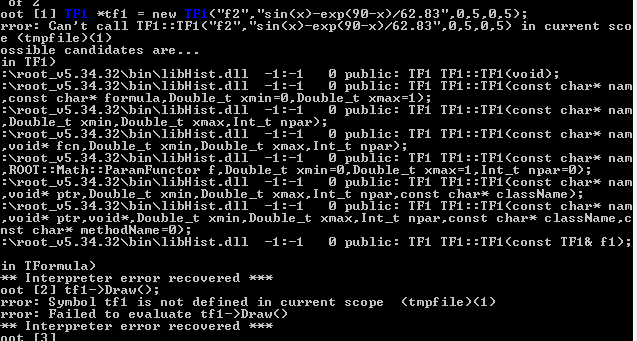# Draw Electric Circuit Graph

I want to draw this function but I have no idea how I can draw it
`Vmax*sin(theta)-Vmax*e^pi/2-theate/wR*C`
f=60 hz
R=4kiloohm``````TF1 *tf1 = new TF1("tf1", "sin(x)-exp(90.0-x)/62.83", 0.0, 5.0);